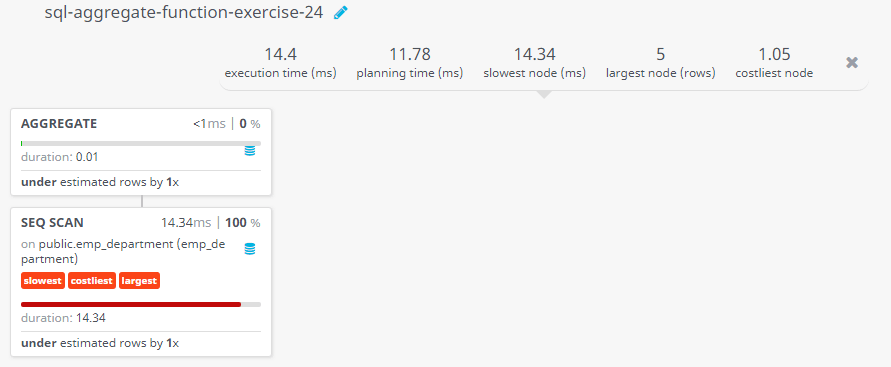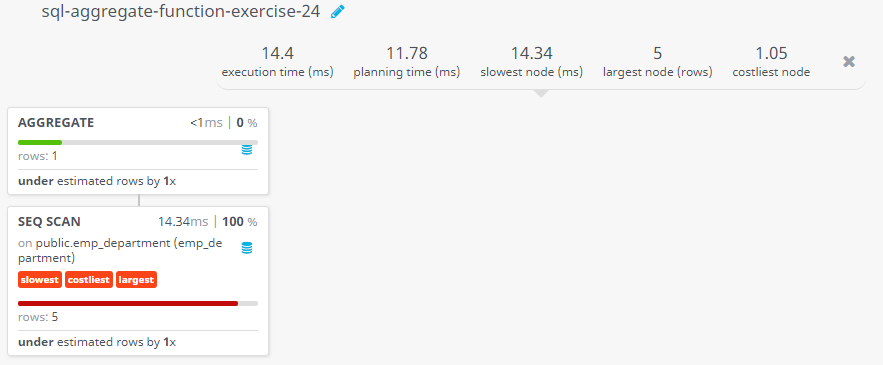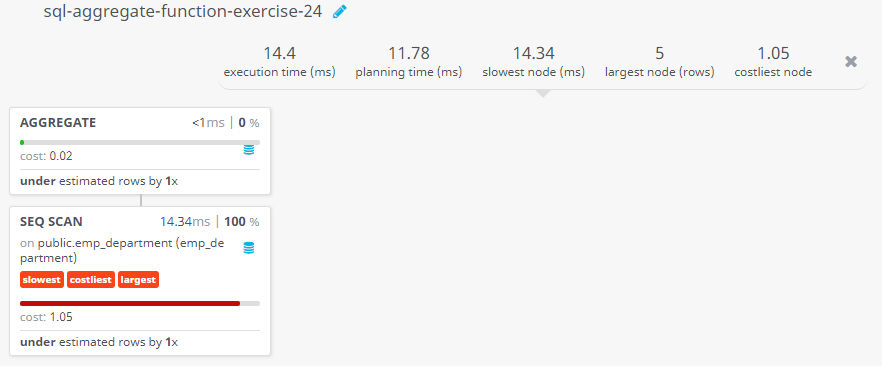﻿ SQL Aggregate Function: Find the sum of the allotment amount of all departments - w3resource# SQL Exercises: Find the sum of the allotment amount of all departments

## SQL Aggregate Functions: Exercise-24 with Solution

Write a query in SQL to find the sum of the allotment amount of all departments.

Sample table: emp_department

Sample Solution:

``````SELECT SUM(dpt_allotment)
FROM emp_department;
``````

Output of the Query:

```sum
450000
```

## Query Visualization:

Duration:Rows:Cost:Have another way to solve this solution? Contribute your code (and comments) through Disqus.

What is the difficulty level of this exercise?

﻿